# Krull's principal ideal theorem

This article gives the statement and possibly, proof, of an implication relation between two commutative unital ring properties. That is, it states that every commutative unital ring satisfying the first commutative unital ring property must also satisfy the second commutative unital ring property
View all commutative unital ring property implications | View all commutative unital ring property non-implications |Get help on looking up commutative unital ring property implications/non-implications
|
This fact is an application of the following pivotal fact/result/idea: Nakayama's lemma
View other applications of Nakayama's lemma OR Read a survey article on applying Nakayama's lemma

## Statement

### Symbolic statement

Let$R$ be a Noetherian and$x \in R$. Let$P$ be a minimal element among prime ideals containing$x$. Then, the codimension of$P$ is at most 1.

### Property-theoretic statement

The property of commutative unital rings of being a Noetherian ring is stronger than the property of being a ring satisfying PIT.

## Proof

### Starting assumptions

In the above setup, we show that if$Q$ is a prime ideal in$R$ contained inside$P$, then the codimension of$Q$, which is the same as the dimension of$R_Q$ is zero. This will show that the codimension of$P$ is at most 1. The crucial thing we shall use is that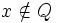$x \notin Q$.

First note that we can replace$R$ by$R_P$, so we may assume that$P$ is a maximal ideal in$R$. We now begin the proof.

### Argument setup

Since$P$ is minimal over$x$, we see that in the ring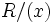$R/(x)$, the ideal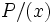$P/(x)$ is the unique maximal ideal of a local ring, and is also a minimal prime ideal. Thus,$R/(x)$ is a local Artinian ring with unique maximal ideal$P/(x)$.

Hence, in$R$, consider the descending chain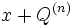$x + Q^{(n)}$, where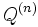$Q^{(n)}$ denotes the$n^{th}$ symbolic power of$Q$. This descending chain stabilizes, so we get:$Q^{(n)} \subset (x) + Q^{(n+1)}$

In particular, we can find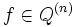$f \in Q^{(n)}$ and$a \in R, g \in Q^{(n+1)}$ such that: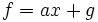$f = ax + g$

This yields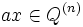$ax \in Q^{(n)}$, so since$x \notin Q$, we get$a \in Q^{(n)}$

### Nakayama's lemma

The above reasoning shows that: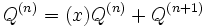$Q^{(n)} = (x)Q^{(n)} + Q^{(n+1)}$

Now consider the module$M = Q^{(n)}/Q^{(n+1)}$. The above equation yields that:$M = (x)M$

But since$x \in P$, we see that$x$ is in the Jacobson radical of$R$, so Nakayama's lemma yields that$M = 0$. Thus$Q^{(n)} = Q^{(n+1)}$.

We now apply Nakayama's lemma in the localization at$Q$, to conclude that: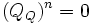$(Q_Q)^n = 0$

This yields that$R_Q$ isa zero-dimensional ring.